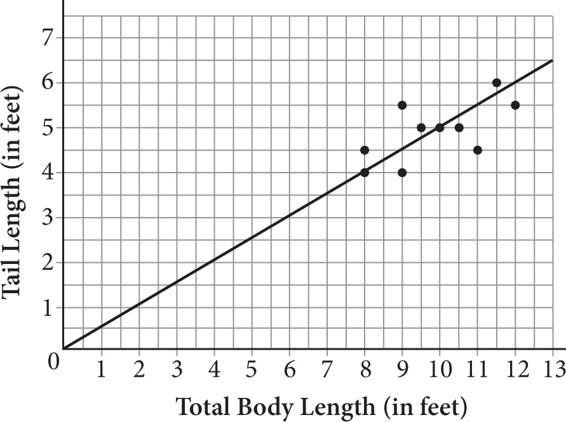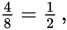# SAT Math Multiple Choice Question 391: Answer and Explanation

### Test Information

Question: 391

1.The Florida Department of Wildlife caught and tagged 10 adult female alligators as part of an effort to protect this endangered species. They took various measurements and readings related to body size and health. The total body length is plotted against the tail length in the scatterplot shown above, along with a line of best fit. Which of the following equations best models the data?

• A. y = 0.5x
• B. y = 2x
• C. y = 0.4x + 1
• D. y = 0.6x - 1

Getting to the Answer: The best-fit line begins at the origin, which means the y-intercept is 0 (the b in the equation y = mx + b), so you can eliminate C and D. Now, find the slope of the line. Between (0, 0) and (8, 4), the line rises 4 units and runs 8 units, so the slope iswhich is equivalent to 0.5. This means (A) is correct.